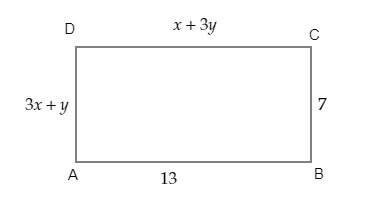"
">

# Find the values of $x$ and $y$ in the following rectangle."

Given:

$ABCD$ is a rectangle in which $AB=13, BC=7, CD=x+3y$ and $AD=3x+y$.
To do:

We have to find the values of $x$ and $y$ in the given rectangle.

Solution:

We know that,

Opposite sides are equal in a rectangle.

Therefore,

$AB=CD$

$13=x+3y$

$x+3y-13=0$....(i)

$BC=AD$

$7=3x+y$

$3x+y-7=0$

$y=7-3x$....(ii)

Using $y=7-3x$ in equation (i), we get,

$x+3(7-3x)-13=0$

$x+21-9x-13=0$

$-8x+8=0$

$8x=8$

$x=\frac{8}{8}$

$x=1$

Using $x=1$ in equation (ii), we get,

$y=7-3(1)$

$y=4$

The values of $x$ and $y$ are $1$ and $4$ respectively.

Updated on: 10-Oct-2022

24 Views